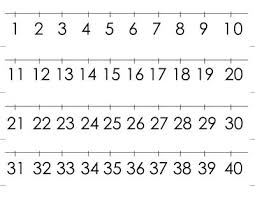# Probability 68594

What is the probability that any two-digit number
a) is divisible by five
b) is it not divisible by five?

p1 =  0.2
p2 =  0.8

### Step-by-step explanation:Did you find an error or inaccuracy? Feel free to write us. Thank you!

Tips for related online calculators
Need help calculating sum, simplifying, or multiplying fractions? Try our fraction calculator.